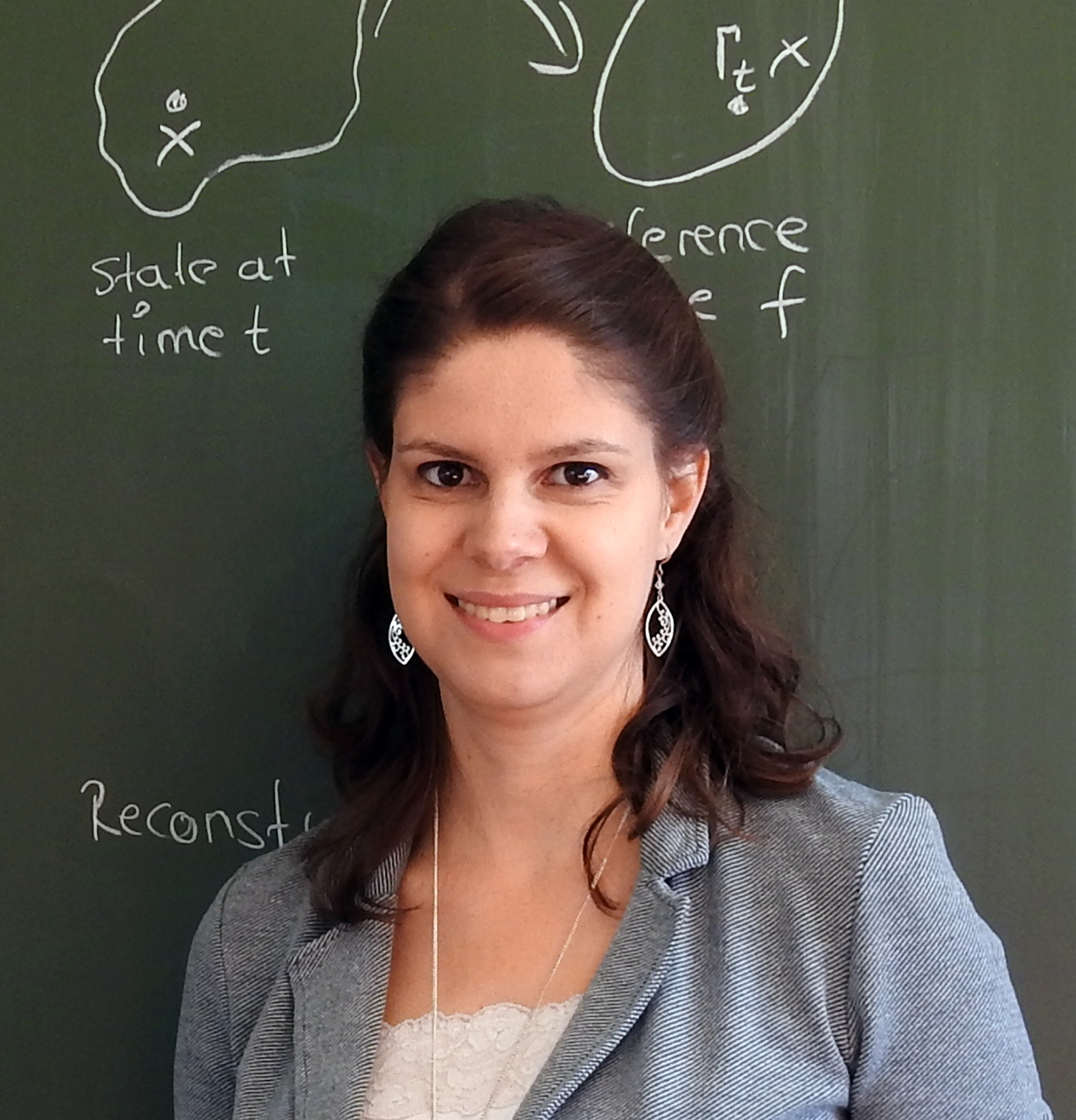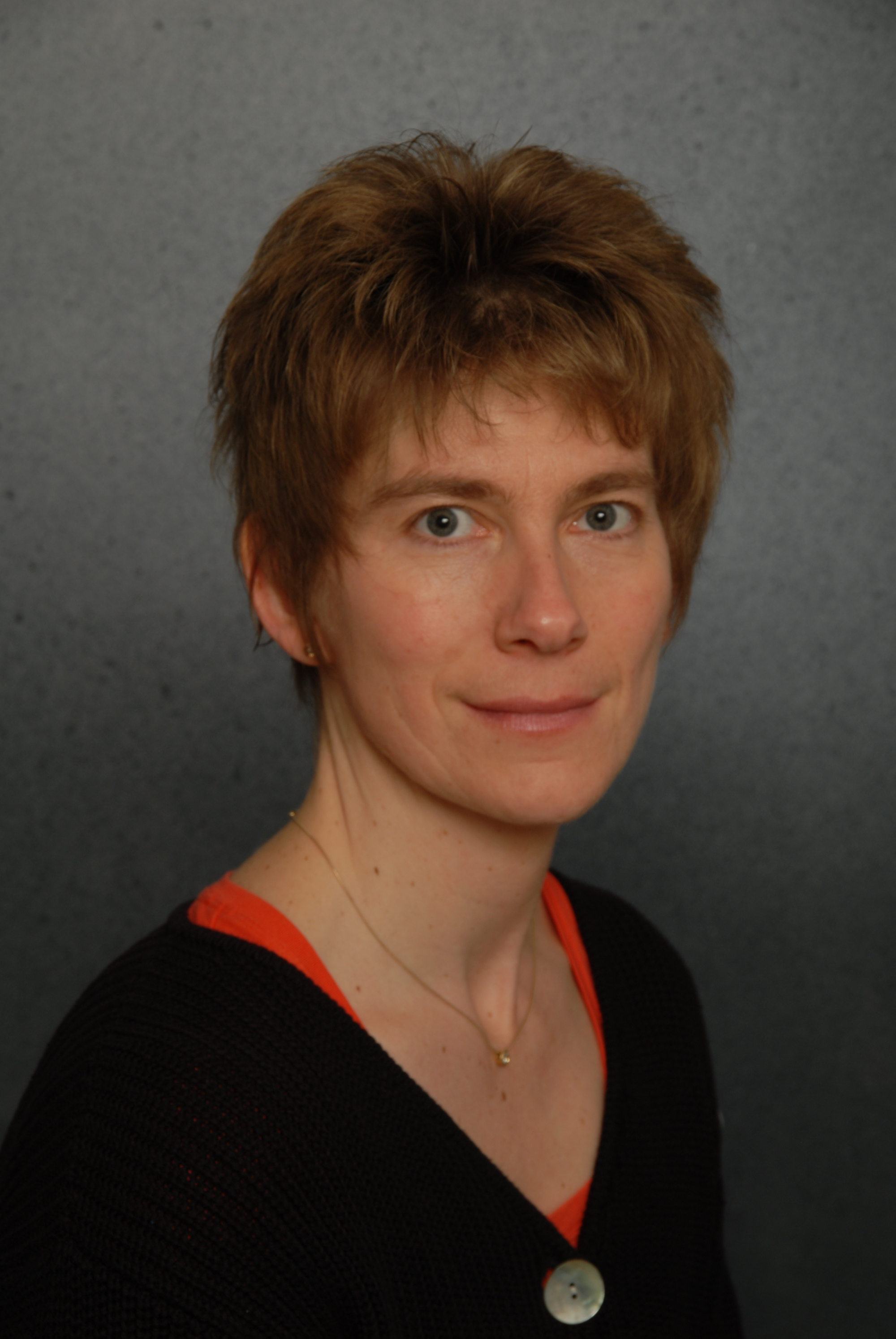Institute of Mathematical Methods in Engineering, Numerical Analysis and Geometric Modeling

Optimal decisions in complex systems with mathematical guarantees

Our institute covers a wide range of topics concerning the mathematical modeling, optimization and control of real-world systems how they emerge in natural sciences, engineering or economics. The chair of Optimization and Inverse Problems focuses on developing methods for choosing optimal decisions in complex systems or for detecting the causes of observed phenomena in mathematically described systems. Research and teaching at the chair for Mathematical Systems Theory is devoted to investigating and developing mathematical methods for the optimal and robust control of dynamical systems.

ContactCarsten W. Scherer

Professor Dr.

Head of Institute and Chairholder mst / Erasmus-Coordinator Dep. of Mathematics

[Photo: Uni Stuttgart]